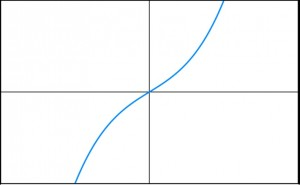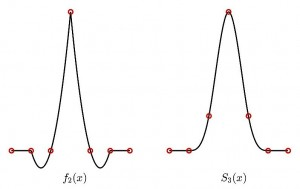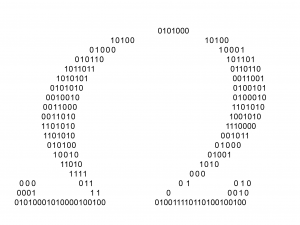# What’s your favourite function? Part II

We have received loads of feedback from people telling us their favourite functionA few weeks ago on this blog we featured some of the Chalkdust team’s favourite functions. Since then we have received loads of feedback from people telling us their favourite function, so in part II of this blog we’re featuring some of the best of the contributions we have received. Our reddit thread got many other excellent contributions, from the Cantor function to the zeta function, and there was a heated debate about whether the Dirac delta function is actually a function (it is).

### The Bessel Function (Ian Stewart)First up is eminent maths populariser, Professor Ian Stewart. Last week we had the privilege of interviewing him for Chalkdust Issue 3, and  the chance to ask him about his favourite function.  His immediate response was the Bessel function. He thinks “they are fascinating because they turn up in all sorts of problems in circular symmetry.” They were first introduced to him at school through a popular maths book by W W Sawyer, which accomplished the task of introducing hypergeometric functions to a general audience. Sawyer described Bessel functions as “like trigonometric functions, but much more interesting”, which intrigued Ian Stewart. He appreciates them because they have all sorts of interesting applications in many different fields: they are connected to representation theory of groups in addition to coming up in optics and astronomy; in fact Bessel himself was an astronomer. Ian Stewart has in the past conducted research involving Bessel functions, looking at a particular type of spiral formation in nature. The proposed hypotheses at the time were that these were Archimidean spirals or involute spirals. Instead Stewart found they were in fact parametrized by a certain combination of Bessel functions.

Look out for the rest of our fascinating interview with Ian Stewart, coming up next year in Issue 3 of Chalkdust!

Next up is Luciano, who has a challenge for you:

### The Taboo Cubic (Luciano Rila)

Before you read this, please sketch a cubic graph. I bet you chose a cubic with two stationary points. Possibly a cubic with one stationary point but you would be in a minority here. I am still to meet someone who would sketch a cubic with no stationary points (I’ll buy you a coffee if you did). Sadly they seem to have vanished from our collective mathematical conscience or at least from our classroom. They are beautiful though, such a simple shape, and I love that their gradient function is a quadratic with complex roots. As we never talk about them, I call them The Taboo Cubic.David Silvester got in touch to suggest an improvement on Matthew Scroggs’s nomination of the linear hat function from Part I. I agree with him, this is far better than the linear hat function:

### Piecewise Quadratic Hat Function (David Silvester)

My favourite function is the piecewise quadratic hat function.$$f_2(x)= \begin{cases} 0 &\mbox{if } x \leq x_{i-2}, \\ {(x-x_{i-1})(x-x_{i-2})\over (x_i-x_{i-1})(x_i-x_{i-2})} &\mbox{if } x_{i-2} \leq x < x_i ,\\ {(x-x_{i+1})(x-x_{i+2})\over (x_i-x_{i+1})(x_i-x_{i+2})} &\mbox{if } x_i \leq x < x_{i+2} ,\\ 0 &\mbox{if } x\geq x_{i+2} .\end{cases}$$

The quadratic hat function is more attractive than the linear hat function (highlighted in the last issue). It is also more useful for practical finite element applications like computing the deflection of an elastic structure when loaded (such as a suspension bridge or a tall building), or computing the motion of a fluid flowing in an axisymmetric pipe. The flow profile that can be observed coming out of a tap is called Poiseuille flow—it can be represented exactly using a small set of quadratic hat functions defined over a radial cross section of the pipe.

The quadratic hat function is also a little rough around the edges (because it does not have a derivative at the joins). The cubic B-spline function $S_3(x)$ is a smooth relative. Cubic spline functions are used everyday in computer graphics and drawing applications where more smoothness is needed. It is just a little too smooth for my taste.

Chris Smith sent us this contribution via Twitter:

### Super-Logarithm (Chris Smith)

The Super-Logarithm function is ok but hard work…it can be a bit of a ‘slog’ to use…

Although in all seriousness the super-logarithm is actually a pretty interesting function. Chris is correct that it is hard to use, check out its intimidating recursive definition on Wikipedia….

### Chaitin’s Constant/ Omega Number (Mike Lynch)My favourite function is usually referred to as Chaitin’s constant or omega number. For a computer program F the omega number is an infinite sum over all of its valid inputs (p):

$$\Omega_F=\sum_{p\in P_F }2^{-p}$$

Omega can be interpreted as the probability that the program F will eventually terminate, rather than go into an infinite loop: computing this is equivalent to solving the halting problem, which was shown to be undecidable by Alan Turing. This gives the omega numbers the perversely intriguing quality they cannot be computed, even in principle. Some properties of all omega numbers have been proven – they converge to a value between 0 and 1, their digits are random, and evenly distributed, they are all transcendental – but only for very simple programs can the first few dozen digits be calculated.

Rob Beckett’s favourite is a classic:

### $e^x$ (Rob Beckett)

My favourite function is the exponential function $f(x)=e^x$. This is primarily because $\frac{d}{dx}(f(x))=e^x$, in other words the function is growing at a rate which is equal to its current size. This is a really interesting property which comes from the fact that $e=\lim\limits_{n\rightarrow \infty}{(1+\frac{1}{n})^n}$. Just like pi, the number $e$ has a special property. It is the base rate of growth which means it crops up whenever things are growing or decaying continuously and exponentially. This exponential function, and variations, appear when considering bacterial growth rates, populations, radioactive decay and can be seen in the Black-Scholes formula that is used in the financial market.

This contribution from Chris Budd is certainly something to think about:

### Unevaluable function (Chris Budd)

My favourite function is something like the following:

$$f(x) = \begin{cases} 1 &\mbox{ if Father Christmas exists} \\ 0 &\mbox{otherwise. } \end{cases}$$It is nice because (like many functions) it is perfectly well defined, but we cannot evaluate it…

Stuart Price has a fantastic example of a really simple function which can exhibit incredibly interesting and complicated behaviour:

### Logistic map (Stuart Price)

The logistic map refers to the family of functions $f_k(x)=kx(1-x)$. I have chosen this as my favourite function as it was my first introduction to chaos theory when I was an A level student. It also appealed to me as I was learning computer programming at the time and it was satisfying to be able to produce my own bifurcation diagram for the parameter space. This model gained popularity through the work of mathematical biologist Robert May published in the 1970s.A significant amount of interesting behaviour is exhibited by this function, and much of it can be analysed with relatively straightforward algebra. The variable $x$ lies between zero and one and represents, as a proportion, the population of a species. In this context the mapping is interpreted as a discrete model with $x_{n+1}=kx_n(1-x_n)$.
It turns out that for values of $k\in[0,1]$ the iterates will converge to zero indicating extinction of the species. However, for $k\in[1,3]$ the long-term population will approach a fixed value, $\frac{k-1}{k}$. For values of $k$ greater than 3, we see what is called a “period doubling cascade’’. The population oscillates between two values, then four, eight, sixteen… ultimately resulting in chaotic behaviour for $k\approx 3.57$ and beyond. Beautifully, however, for a range of values beginning at $k=1+\sqrt{8}$ there is an oscillation between three values, which then  exhibits its own period doubling cascade. There is also a fundamental connection between the mathematics of this model and the Mandelbrot set. Although they parameterise the family of quadratics in slightly different ways, the line of symmetry of the Mandelbrot set exhibits the same dynamical behaviour as the logistic map.And finally, we also had an interesting function from a German contributor, clearly inspired from one of the choices from part I:

### Funky Funktion (Mattheas Recht)

Let $\mathbb{S}$ be the set of all strings of letters from the Roman alphabet. Then meine lieblingsfunktion ist the map $F: \mathbb{S}\rightarrow \mathbb{S}$ such that
\begin{align*}
F(x)=\begin{cases} \textrm{funk}x &\textrm{ if } x=\textrm{tions} \\
x &\textrm{ otherwise} \end{cases}
\end{align*}
Why do I like this funktion? Because it is the funktion which puts the funk into funktions! Funky.Matt is a PhD student at UCL, working in the fields of general relativity and cosmology.

## 3 thoughts on “What’s your favourite function? Part II”

1. Pingback: My favourite function… | rbeckettyd

2. Pingback: My favourite function… | rbeckettyd